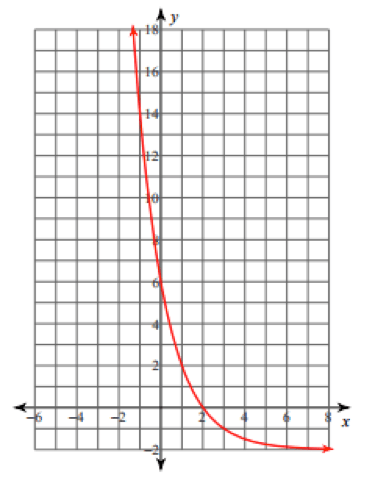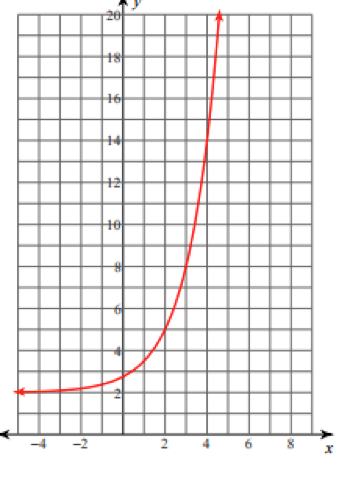# FNGR 1 | Lesson 2 | Practice (Assess)# Assess

Identify each of the following as exponential growth or decay.

1.2.3.4.5.  $$f(x)=700(0.75)^x$$

6.  $$f(x)= \dfrac{2}{3} \cdot 4^x$$

Identify the following problems as growth or decay and then solve them.

1. The half-life of caffeine is 5.75 hours.   Janine drank a cup of coffee at 7:30 am with 150 mg of caffeine, how much caffeine remains in her system at 10 am?
2. \$10,000 is going to be invested.  Would it be better to invest it in an account that provides interest quarterly or daily, if the interest rate is 3% over 10 years?

Check solutions here.